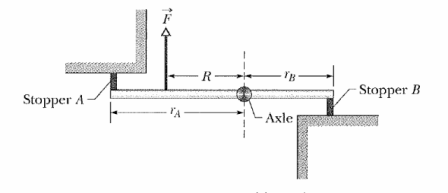# The force it applies.Figure above is an overhead view of a rigid rod that turns about a vertical axle until the identical rubber stoppers A and B are forced against rigid walls at distances $r_{A} = 7$ $cm$ and $r_{B} = 4$ $cm$ from the axle. Initially the stoppers touch the walls without being compressed. Then force $\vec{F}$of magnitude $220$ $N$ is applied perpendicular to the rod at a distance $R = 5$ $cm$ from the axle. Find the magnitude of the force compressing Stopper A.

Liked it? try some more

×